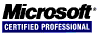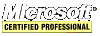## .NET What's New or Changed in C# 7

Improvements to C# are discussed at https://github.com/dotnet/csharplang. Here, you can find proposals to the language and submit your own.

#### async Main

In C# 7.1, the main method can be a `Task` using `await` and `async`.

``````class Program
{
{
await AsyncMethod();
}
}
``````

#### Expresssion-Bodied Members

Constructors, Destructors and accessors can be expresson-bodied members.

``````class Business
{
private string _name;

// expression-bodies get & set accessors
public string Name { get => _name; set => _name = value ?? ""; }

// expression-bodied constructor
public Business(string name) => Name = name;

// expression-bodied destructor
}
class Program
{
static void Main(string[] args)
{
Console.WriteLine(bus.Name);
}
}
``````

#### New out Variables

`out` variables can be declared on use.

``````string s = "123";
if(int.TryParse(s, out int num))
{
// do something with num
num++;
}
``````

#### New in Parameters

In C# 7.2, `in` function parameters cannot be modified and, like `ref`, are passed by reference thereby avoiding a copy operation.

``````string str = "abc";
void DoSomething(in string s)
{
// s can be accessed but not modified
}
``````

#### Tuples

Tuples allow the combining of objects of different types. Previously, tuples required the use of the `Tuple` class. Now, in C# 7, tuples are part of the language. In C# 7.1, tuples are further extended by automatically inferring the tuple name.

``````var tup1 = (str: "this is a string", num: 123);
int num = tup1.num;
string str = tup1.str;
double price = tup2.Price;
``````
``````// in C# 7.1, tuples automatically infer the tuple name
double price = tup2.Price;
``````

As of C# 7.3, tuples now support `==` and `!=`. These comparisons short-circuit meaning that they stop comparing when further evaluation is not important or does not affect the outcome. Read more about short-circuiting under operators.

#### Deconstructors

Tuples can be deconstructed (as well as user defined objects).

``(string name, int age) = ("Jane", 35);``

#### Non-trailing Named Arguments

In C# 7.2, arguments need not be named when trailing named arguments.

``````string str = "10";
if(int.TryParse(s: str, out int num)) // this will not generate an error in C# 7.2
{
num *= 100;
}
``````

In C# 7.2, it is possible to declare a `struct` as `readonly` so that the compiler makes sure that the struct goes unchanged.

``````public readonly struct RightTriangle
{
public double leg1 { get; }
public double leg2 { get; }

public RightTriangle(double leg1, double leg2)
{
this.leg1 = leg1;
this.leg2 = leg2;
}

public double Hypontenuse() => Math.Sqrt(leg1 * leg1 + leg2 * leg2);
}
``````

#### private protected

`private protected` allows access only if the class using these keywords derives from the base class and is in the same assembly.

``````// now there are digit separators to help make these and other number literals more readable
uint hex = 0x_FF_1E_23_56
``````

#### Target-Typed default

In C# 7.1, a default literal is now defined to help save typing time when the type has a long name.

``System.Collections.Generic.IEnumerable<string> iestr = default;``

#### Local Functions

A local function can be declared within a method. This is not a lambda expression.

``````public void SomeMethod()
{
int multiply(int a, int b) => a * b; // a local function

int x = multiply(3, 4);
}
``````

#### Pattern Matching with switch and is

`switch` and `is` are enhanced with the `var` pattern, the type pattern and the `const` pattern.

``````object obj = null;
if(obj is Square s)
{
s.Length = 100;
}
if (obj is 30)
{
;
}
if(obj is var v)
{
Console.WriteLine(v);
}
switch(obj)
{
case 33:
break;
case Square sq when sq.Length > 0:
sq.Length *= 2;
break;
case Square sq:
sq.Length = 40;
break;
case var va:
Console.WriteLine(va);
break;
}
``````

#### ref Locals and Returns

Now it's possible to use `ref` with local variables and the return type. A `ref` local is basically a pointer.

``````class Temp
{
private int[] nums = { 0, 8, 4, 12, 1, 5 };
private ref int GetPointerToNumber(int i)
{
return ref nums[i];
}
public void Run()
{
ref var idx3 = ref GetPointerToNumber(3);
idx3 <<= 1; // multiply by 2, nums == 24
}
}
``````

In C# 7.2, using `readonly`, the caller gets a reference to the data but is not allows to change it.

``````class Temp
{
private int[] nums = { 0, 8, 4, 12, 1, 5 };
private ref readonly int GetPointerToNumber(int i)
{
return ref nums[i];
}
public void Run()
{
ref readonly var idx3 = ref GetPointerToNumber(3);
idx3 <<= 1; // cannot do this, idx3 is readonly
}

}
``````

#### Digit Separators

The underscore (_) serves as a digit separator.

``````// now there are digit separators to help make these and other number literals more readable
uint hex = 0x_FF_1E_23_56
``````

#### Binary Literals

Totally new are binary literals.

``````uint bin = 0b1010000100000001
// now with digit separators
uint bin2 = 0b_10100001_00000001
``````